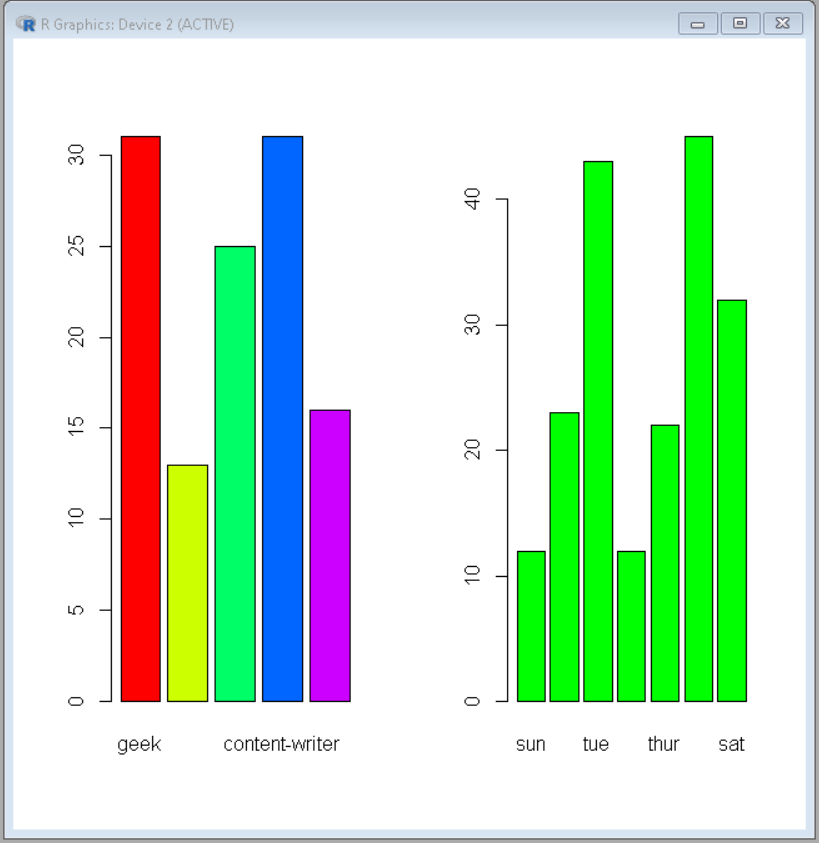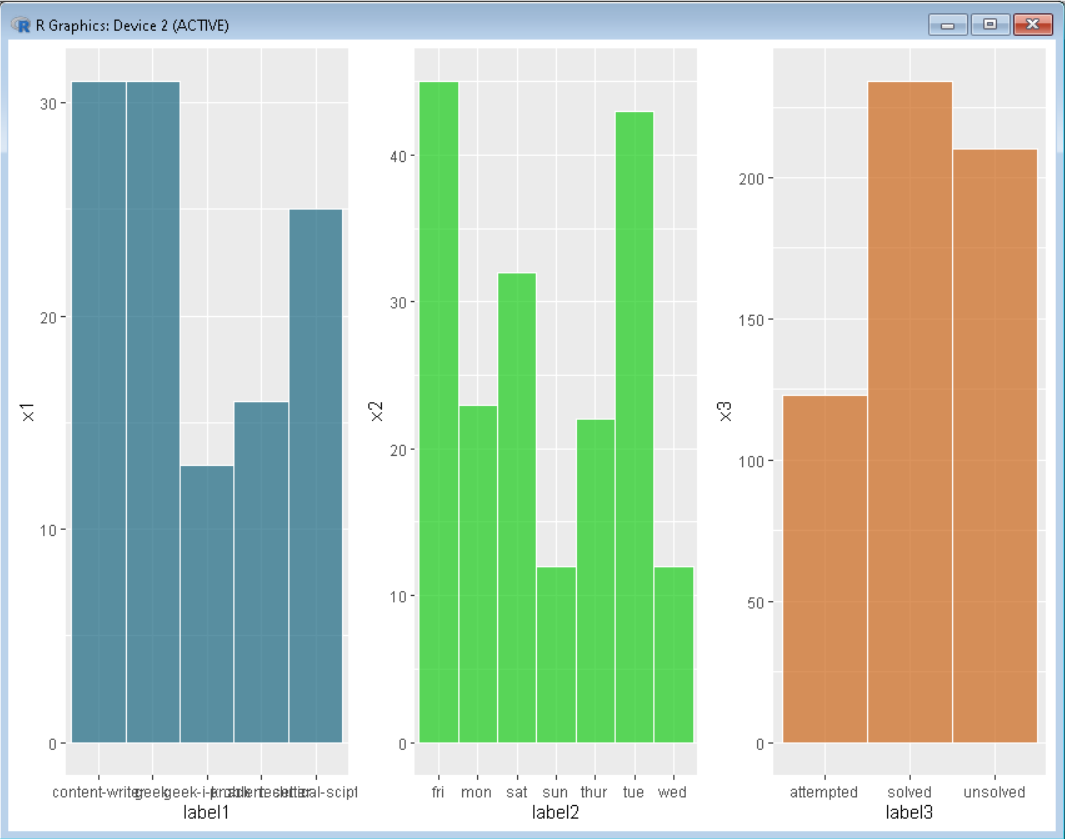# Side by Side bar charts in R

• Last Updated : 01 Feb, 2022

In this article, we will discuss how to draw Bar Charts side by side in R Programming Language.

## Method 1: Using par() function

To draw plots side by side par() function is used.

Syntax:

par(mfrow, mar, mgp, las)

Parameters:

• mfrow- A numeric vector of length 2, which sets the rows and column in which frame has to be divided.
• mar – A numeric vector of length 4, which sets the margin sizes in the following order: bottom, left, top, and right.
• mgp – A numeric vector of length 3, which sets the axis label locations relative to the edge of the inner plot window.
• las – A numeric value indicating the orientation of the tick mark labels and any other text added to a plot after its initialization.

The plots are drawn normally and independent of others. For drawing them side-by-side pass the number of rows and columns as if a grid is being defined.

Example: Plotting bar plots side by side using basic R

## R

 `# Define data-set columns` `x1 <- ``c``(31,13,25,31,16)` `x2 <- ``c``(12,23,43,12,22,45,32) `   `label1 <- ``c``(``'geek'``,``'geek-i-knack'``,``'technical-scripter'``,` `            ``'content-writer'``,``'problem-setter'``)` `label2 <- ``c``(``'sun'``,``'mon'``,``'tue'``,``'wed'``,``'thur'``,``'fri'``,``'sat'``)  `   `# set the plotting area into a 1*2 array` `par``(mfrow=``c``(1,2))    `   `# Draw the two bar chart using above datasets` `barplot``(x1, names.arg = label1,col=``rainbow``(``length``(x1)))` `barplot``(x2, names.arg = label2,col =``"green"``)`

Output:## Method 2: Using ggplot2

In this grid.arrange() is used to arrange the plots on a frame.

Syntax:

grid.arrange(plot, nrow, ncol)

Parameter:

• plot- ggplot2 plot which we want to arrange
• nrow- Number of rows
• ncol- Number of columns

Here, plots are drawn normally and independently. Then, the function is called with these plots and the number of rows and columns in a way that a grid is being defined.

Example: Plotting multiple plots side by side using ggplot.

## R

 `# Define data-set columns` `x1 <- ``c``(31,13,25,31,16)` `x2 <- ``c``(12,23,43,12,22,45,32) ` `x3 <- ``c``(234,123,210)`   `label1 <- ``c``(``'geek'``,``'geek-i-knack'``,``'technical-scripter'``,` `            ``'content-writer'``,``'problem-setter'``)` `label2 <- ``c``(``'sun'``,``'mon'``,``'tue'``,``'wed'``,``'thur'``,``'fri'``,``'sat'``)  ` `label3 <- ``c``(``'solved'``,``'attempted'``,``'unsolved'``)`   `# Create data frame using above ` `# data column` `data1 <- ``data.frame``(x1,label1)` `data2 <- ``data.frame``(x2,label2)` `data3 <- ``data.frame``(x3,label3)`   `# set the plotting area into a 1*3 array` `par``(mfrow=``c``(1,3))    `   `# import library ggplot2 and gridExtra` `library``(ggplot2)` `library``(gridExtra)`   `# Draw the three bar chart using above datasets` `plot1<-``ggplot``(data1, ``aes``(x=label1, y=x1)) + ` `geom_bar``(stat=``"identity"``, width=1, color=``"white"``,` `         ``fill=``rgb``(0.1,0.4,0.5,0.7))`   `plot2<-``ggplot``(data2, ``aes``(x=label2, y=x2)) + ` `geom_bar``(stat=``"identity"``, width=1, color=``"white"``,` `         ``fill=``rgb``(0.1,0.8,0.1,0.7))`   `plot3<-``ggplot``(data3, ``aes``(x=label3, y=x3)) +` `geom_bar``(stat=``"identity"``, width=1, color=``"white"``, ` `         ``fill=``rgb``(0.8,0.4,0.1,0.7))`   `# Use grid.arrange to put plots in columns` `grid.arrange``(plot1, plot2, plot3, ncol=3)`

Output:My Personal Notes arrow_drop_up
Recommended Articles
Page :